# Magnetic Field

Magnetic Fields is the region around a magnet in which it exerts a force on other magnetic material. The magnetic field of the earth protects us from harmful solar radiation as well as the production of electricity for homes is also an application of the Magnetic Field. Thus everybody should know about the basics of it. In this article, we will explore the concepts of magnetic fields in a well-detailed manner. Other than that we will also learn about the magnetic field line, its properties, application, and intensity as well. So, let’s explore the fascinating world of magnetic fields and learn how they shape our lives.

## Magnetic Field Definition

According to the definition of the magnetic field, it is the region around a magnet or current-carrying conductor around which the force of magnetism can be observed.

It is a vector quantity and is generated by moving charges. Magnetic forces and Electric forces are part of one of the four fundamental forces of nature. Electric and Magnetic forces interplay with each other to form electromagnetic forces which are an essential part of our modern engineering infrastructure and development.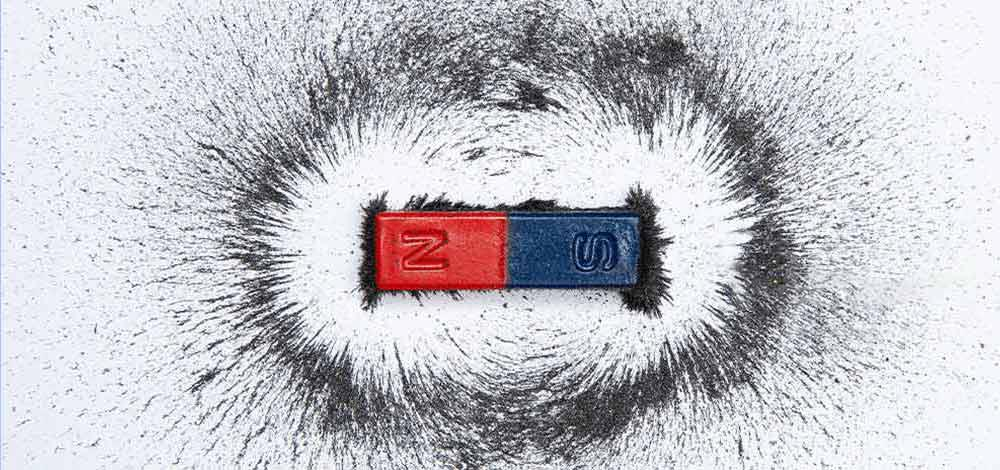### History of Magnetic Field

The research about magnetic fields was first observed in the 13th century when a French scholar Petrus Peregrinus de Maricourt used iron needles to map out the magnetic field lines around a sphere magnet. The points where these lines crossed, he names them poles. He stated that magnets have poles – North and South. Centuries later William Gilbert claimed in his experiments that the earth is a magnet. After that observation, during the 18th and 19th centuries, several theorists and researchers came up with theories around attraction and repulsion in magnetic poles. English scientist Faraday discovered that changing magnetic fields produce electricity and gave the theory of electromagnetic induction. Later, James Maxwell published theories on electricity and magnetic field intensities and their interplay. They came to be known as Maxwell’s Equation and are an integral part of modern engineering.

## Unit of Magnetic Field

The SI unit for the magnetic field is the tesla (T).

• B-Field: It is the magnetic field defined by the force it exerts on a moving charged particle.
• H-Field: It is defined as the magnetic field within a material, rather than in a vacuum.

B-Field is measured in Tesla (T) while H-Field is measured in Ampere/meter (A/m). In the CGS system, the unit of measurement for the B-field is the gauss (G), (1 T = 10000 G), while the H-field is measured in Oersted (Oe) (1 Oersted = 1000/4π A/m ≈ 79.577 A/m).

## Illustration of Magnetic Field

A Magnet is a material that has the ability to produce a magnetic field which creates magnetic field lines, the field force is able to attract and repel other ferromagnetic materials like iron, nickel, etc. The magnetic field can be illustrated using two possible ways – Magnetic Field Vector and Magnetic Field Lines.

### Magnetic Vector Field

The magnetic field is a vector quantity (it has both magnitude and direction), and the direction of the magnetic field is from the north pole to the South Pole. It is a vector field, mathematically which means it is a set of many vectors which points in different directions. The figure below illustrates a magnetic field using a magnetic field vector. The length of these vectors determines the strength of the field at that particular point.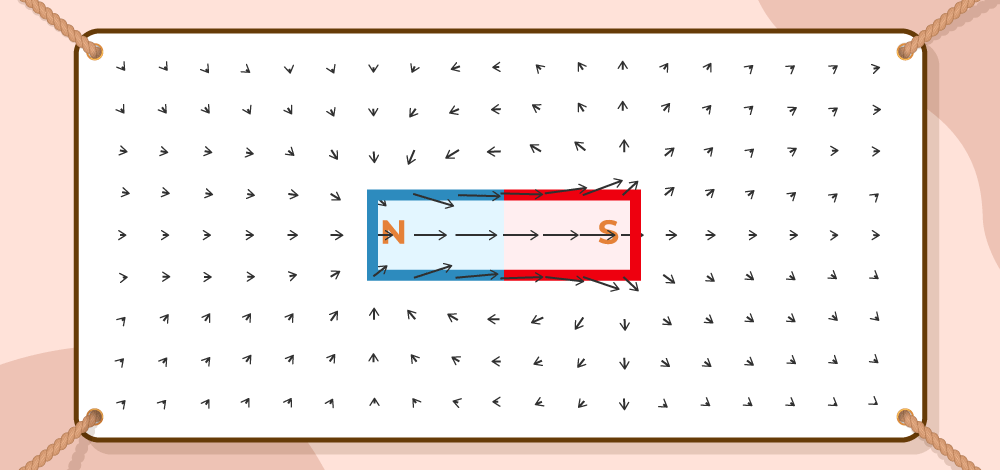## Magnetic Field Lines

Another representation of the magnetic field can be done using the magnetic field lines which are shown in the figure below. The strength of the magnetic field is shown here by the closeness of magnetic field lines. For example, the magnetic field is stronger at the poles because the lines are more crowded in that area.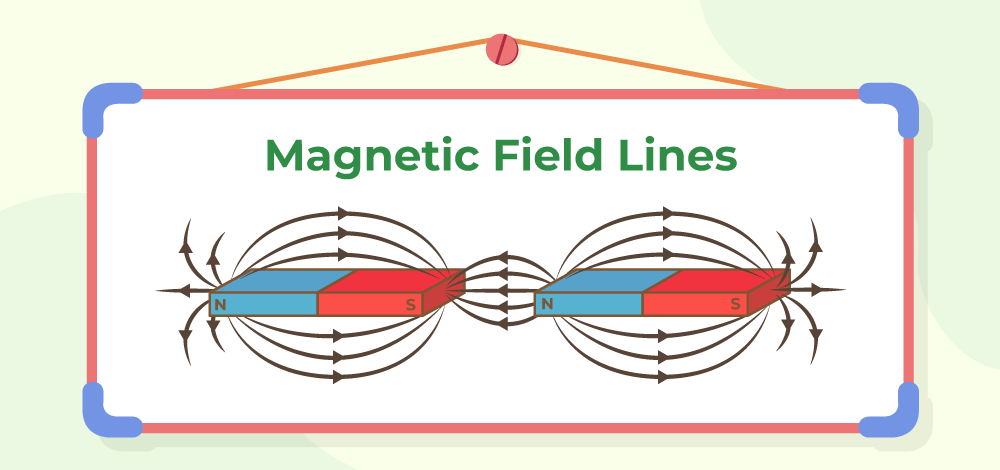### Properties of Magnetic Field Lines

Some of the properties of magnetic field lines are as follows:

• Magnetic Field lines never cross each other.
• Tangent to magnetic field lines gives the direction of the magnetic field.
• The density of field lines is directly proportional to the strength of the field
• Field lines always form a closed loop.
• Field lines emerge from the north pole and terminate at the south pole.
• Inside the magnet, the direction of field lines is reversed, flowing from south to north.
• Magnetic field lines have both direction and magnitude, represented by a vector.
• The magnetic field is stronger at poles due to denser field lines.

## Magnetic Field Intensity

Magnetic field Intensity denotes the strength of the magnetic field. It is represented by H and is a vector quantity. It is defined as the magnetomotive force(MMF) needed to create a flux density B inside a material per unit length of that material. The unit of Magnetic Field intensity is Ampere/Meter and the dimensional formula for the same is given by [M1T-2I-1]. SI unit of Magnetic Field Intensity is Tesla.  A magnetic field of strength One Tesla can also be thought of as the magnetic field producing a force of 1 Newton per ampere of current in each meter of conductor.

H = B/μ – M

here,

• B is the Magnetic Flux Density
• M is Magnetization
• μ is Magnetic Permeability

### Magnetic Effect of Electric Current

When current is passed through a magnetic material, a magnetic field is produced, if a needle is placed near the material, providing the electric charge (moving charges), the needle will deflect. The area to the point where the force is exerted by the magnetic material is called a magnetic field.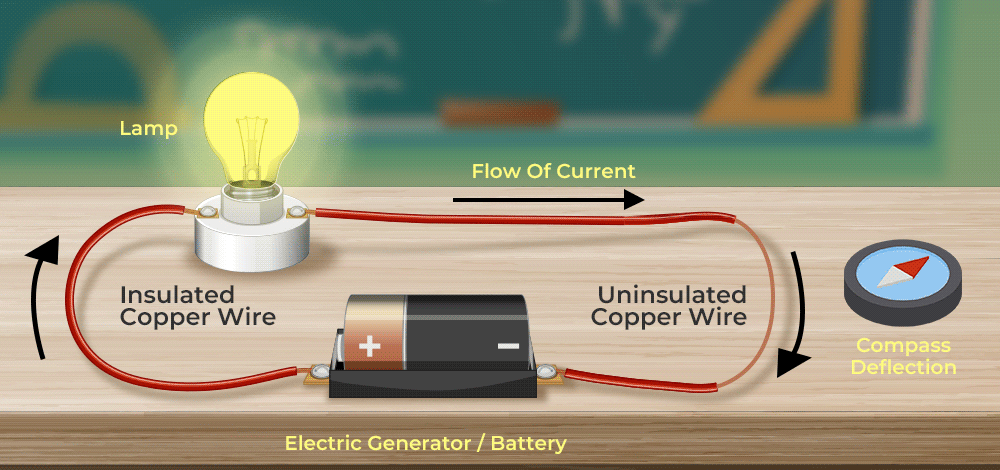## Magnetic Field lines due to Current Carrying Straight Conductor

The magnetic field lines produced by a straight current-carrying conductor is in the form of concentric circles, depending upon the direction of the current, magnetic field lines also change their directions.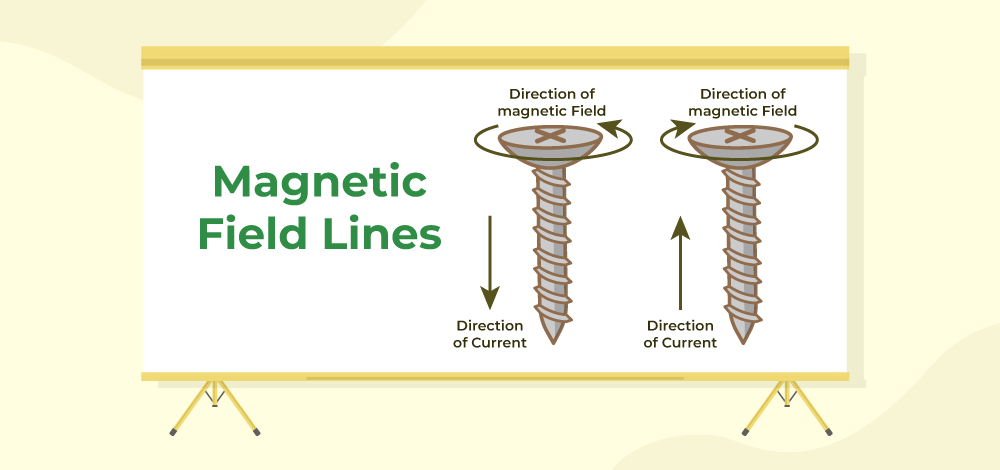The direction of the magnetic field lines can be identified by

• Right-hand Thumb Rule
• Maxwell’s Corkscrew Rule

Let’s understand these rules in detail as follows:

### Right-Hand Thumb Rule

According to Right- Hand Thumb Rule, If the right hand’s thumb is placed in the direction of the moving current, then the direction of the fingers shows the direction of magnetic field lines, Similarly, if the thumb is placed in the direction of the magnetic field lines, then the direction of current is shown by the curled fingers’ direction.

### Maxwell’s Corkscrew Rule

According to Maxwell’s Corkscrew rule, A screw can tell the direction of the magnetic field lines. If the screw is moved forward, the direction of rotation shows the direction of magnetic field lines while the direction of the movement of the screw shows the direction of current and Vice-Versa.

## Magnetic Field lines due to Current through a Circular Loop

The magnetic field lines will create the same pattern as they created in the case of a straight current-carrying conductor, That means, the field lines will be concentric in nature, but based on the direction of the current, the direction of field lines will change as well. For example, in a circular loop, imagine two places, one where the current goes upward and one where the current goes downward, according to the right-hand thumb rule, in the first case, the field lines must be anticlockwise in nature, and in the second case, the field lines must be clockwise in nature.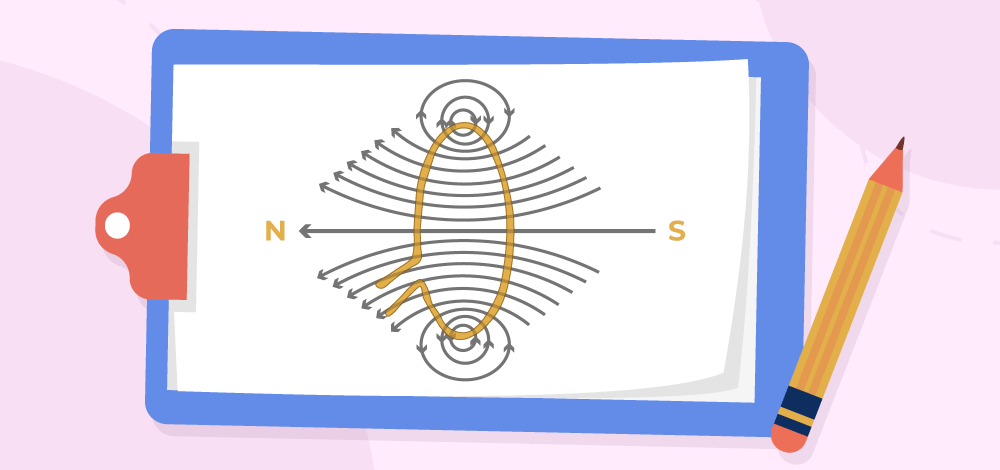## Magnetic Field lines due to Current in Solenoid

A solenoid is a helical coil which upon giving current becomes an electromagnet and forms the North and South Pole and due to moving charges, it creates Magnetic field lines. The field lines created by the solenoid look similar to the field lines created by a simple Bar magnet.## Force on a current-carrying conductor placed in a Magnetic Field

Imagine a wire (conductor) is placed under a magnet and current is passed through the wire, due to the moving electric charges in a magnetic field, a force is applied to the conductor that moves it in a certain direction, this phenomenon was given by Michael Faraday. The direction of the force on the conductor is determined by Fleming’s left-hand rule.

### Fleming’s Left-hand Rule

According to Fleming’s left-hand rule, the direction of force can be determined with the help of the left hand’s thumb, index finger, and middle finger. The thumb represents the Force, The index finger shows the direction of the magnetic field lines and the middle finger shows the direction of the current. Therefore, if the middle finger and index finger are kept in the direction of current and magnetic field lines respectively, the thumb will represent the direction of force produced on the current-carrying conductor.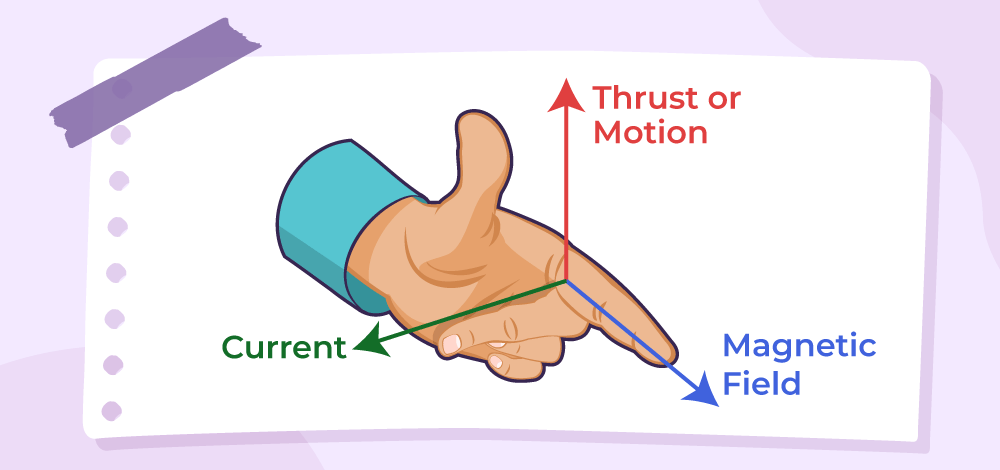## Difference between Magnetic Field and Electric Field

There are some key differences between Magnetic Field and Electric Field, which are listed in the following table:

## Electromagnet

An electromagnet is a type of magnet formed due to the current moving and passing through it, it is a type of temporary magnet and is mostly seen as a coil wrapped over a soft iron core (for example, solenoid).

### Properties of Electromagnet

• The electromagnets are not permanent magnets, they are temporary in nature.
• The strength of the magnetic force can be altered by varying currents.
• Electromagnets are made with soft iron cores.

Note: When current is applied on a ferromagnetic material to produce magnetic field lines, the magnetic force is dependent on the number of magnetic dipole aligned, The amount of current applied is directly proportional to the number of dipoles alignment and the amount of magnetic force can be altered. However, it can only be done up to some point, till the point where saturation reaches and no dipoles can further be available for alignment.

## Sample Questions on Magnetic Field

Question 1: What are the applications of Fleming’s left-hand rule?

Fleming’s left-hand rule is applied in Electric motors (DC motors, induction motors, etc). The basic concept is that the induced current produces force on the conductor which helps in creating motion.

Question 2: What is the difference between a Permanent magnet and an electromagnet?

A Permanent magnet is made up of Hard material, The magnetic field is produced due to magnetization and once the magnetization is lost, the magnet ceases to show magnetic properties since the magnetic dipoles cannot be changed further.

An electromagnet is made up of Soft material, The magnetic field is produced by applying current through it. Once the current stops, the magnetism stops but again develops after applying current again, The dipoles aligned in an electromagnet can be altered again and again.

Question 3: Write down the Properties of Magnetic field lines.

Properties of magnetic field lines

• The magnetic field lines decide the strength of the magnetic field, denser the field lines, stronger the magnetic field.
• They always form closed and continuous loops.
• Magnetic field lines never cross each other, since they do not have two directions.

Question 4: Why are magnetic flux lines important?

Magnetic flux lines are the lines of magnetic field, the tangent at any point to these lines denote the direction of the magnetic field at that particular point. Their density gives us the idea about the strength of the magnetic field in a region. The density of these lines are higher at the poles, that is why poles have very strong magnetic field strength in their vicinity.

Question 5: Why do magnetic field lines vary in density?

Magnetic field lines can vary in density because the field lines start near the magnet, and then they go further away and as they go further away from the magnet, the effect of magnetic force gradually decreases and stops after a point, since the force is decreasing, the density of the magnetic field lines also tend to decrease.

Question 6: List two methods for creating a magnetic field.

Magnetic Field can be generated by following two methods:

1. Magnet Bar, horse-shoe magnet or a round magnet.
2. Moving current carrying condutor, solenoid etc.

Question 7: What causes Earth’s magnetic field?

Magnetic field of earth is generated inside the core of earth due to flow of liquid iron at the centre. Due to these flows, electric current is generated and thus a changing magnetic field is generated which in turn generates more current. This cycle continues and generates a magnetic field around earth.

## FAQs on Magnetic Field

### Q1: Define Magnetic Field.

A magnetic field is a region around a magnet or a moving charged particle that exerts a force on other magnets or moving charges.

### Q2: What causes Magnetic Field?

A magnetic field is caused by the motion of charged particles, such as electrons, in a magnetic field.

### Q3: What is the Unit of Magnetic Field?

The unit of magnetic field is the tesla (T) in the SI system and in CGS system unit of magnetic field is Gauss(G).

### Q4: What is the Difference between a Magnetic Field and an Electric Field?

The key difference between both a magnetic field and an electric field is that a magnetic field is produced by the motion of charged particles, while an electric field is produced by the presence of an electric charge.

### Q5: How do Magnetic Fields affect Materials?

Magnetic fields can cause certain materials, such as iron, to become magnetized, which can then exert magnetic forces on other materials.

### Q6: Can Magnetic Fields be harmful to humans?

Highly intense magnetic fields can be harmful to humans, causing headaches, dizziness, and other health problems. However, the magnetic fields encountered in everyday life are generally not strong enough to cause harm.

### Q7: Define Magnetic Field Lines.

Magnetic field lines are the imaginary lines around the source of the magnet which can be used to represent the direction of the magnetic field at that point.

### Q8: Why do Magnetic Field Lines never Intersect?

Since magnetic field is a vector field, the lines denote the direction of magnetic field. If two magnetic field lines intersect each other, there will be two directions of the magnetic field at a single point which is not possible. Thus, magnetic field lines do not intersect each other.

### Q9: What is Magnetic Field Intensity?

The measure of strength of the magnetic field is called magnetic field intensity and it is denoted by H.